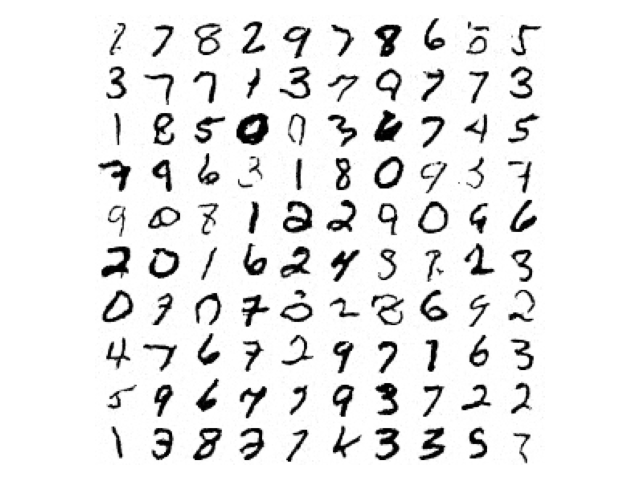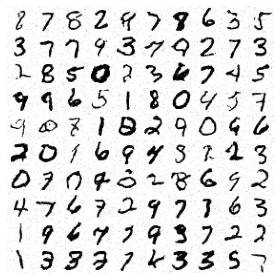# Generative score-based diffusion¤

In this example we train a score-based diffusion as a generative model for MNIST digits.This example:

• Uses the variance-preserving SDE to corrupt the data:

$$y(0) \sim \mathrm{data}\qquad\mathrm{d}y(t) = -\frac{1}{2} β(t)y(t)\mathrm{d}t + \sqrt{β(t)}\mathrm{d}w(t) \qquad\text{for }t \in [0, T].$$

• Trains a score model $$s_\theta$$ according to the denoising objective:

$$\arg\min_\theta \mathbb{E}_{t \sim \mathrm{Uniform}[0, T]}\mathbb{E}_{y(0) \sim \mathrm{data}}\mathbb{E}_{(y(t)|y(0)) \sim \mathrm{SDE}} \lambda(t) \| s_\theta(t, y(t)) - \nabla_y \log p(y(t)|y(0)) \|_2^2$$

• Uses the equivalent ODE for sampling (solved using the Diffrax library):

$$y(1) \sim \mathcal{N}(0, I)\qquad\mathrm{d}y(t) = -\frac{1}{2}β(t) (y(t) + s_\theta(t, y(t)))\mathrm{d}t \qquad\text{for }t \in [0, T].$$

• Uses an MLP-Mixer to parameterise the score model $$s_\theta$$.

Warning

This example will take a short while to run on a GPU.

Reference

@inproceedings{song2021scorebased,
title={Score-Based Generative Modeling through Stochastic Differential
Equations},
author={Yang Song and Jascha Sohl-Dickstein and Diederik P Kingma and
Abhishek Kumar and Stefano Ermon and Ben Poole},
booktitle={International Conference on Learning Representations},
year={2021},
}

import array
import functools as ft
import gzip
import os
import struct
import urllib.request

import diffrax as dfx  # https://github.com/patrick-kidger/diffrax
import einops  # https://github.com/arogozhnikov/einops
import jax
import jax.numpy as jnp
import jax.random as jr
import matplotlib.pyplot as plt
import optax  # https://github.com/deepmind/optax

import equinox as eqx


First let's specify our score-based model $$s_\theta$$, as an MLP-Mixer. We'll use many of the pre-built equinox.nn layers here.

We encode time-dependence in a simple way, by just concatenating it as another channel.

class MixerBlock(eqx.Module):
patch_mixer: eqx.nn.MLP
hidden_mixer: eqx.nn.MLP
norm1: eqx.nn.LayerNorm
norm2: eqx.nn.LayerNorm

def __init__(
self, num_patches, hidden_size, mix_patch_size, mix_hidden_size, *, key
):
tkey, ckey = jr.split(key, 2)
self.patch_mixer = eqx.nn.MLP(
num_patches, num_patches, mix_patch_size, depth=1, key=tkey
)
self.hidden_mixer = eqx.nn.MLP(
hidden_size, hidden_size, mix_hidden_size, depth=1, key=ckey
)
self.norm1 = eqx.nn.LayerNorm((hidden_size, num_patches))
self.norm2 = eqx.nn.LayerNorm((num_patches, hidden_size))

def __call__(self, y):
y = y + jax.vmap(self.patch_mixer)(self.norm1(y))
y = einops.rearrange(y, "c p -> p c")
y = y + jax.vmap(self.hidden_mixer)(self.norm2(y))
y = einops.rearrange(y, "p c -> c p")
return y

class Mixer2d(eqx.Module):
conv_in: eqx.nn.Conv2d
conv_out: eqx.nn.ConvTranspose2d
blocks: list
norm: eqx.nn.LayerNorm
t1: float

def __init__(
self,
img_size,
patch_size,
hidden_size,
mix_patch_size,
mix_hidden_size,
num_blocks,
t1,
*,
key,
):
input_size, height, width = img_size
assert (height % patch_size) == 0
assert (width % patch_size) == 0
num_patches = (height // patch_size) * (width // patch_size)
inkey, outkey, *bkeys = jr.split(key, 2 + num_blocks)

self.conv_in = eqx.nn.Conv2d(
input_size + 1, hidden_size, patch_size, stride=patch_size, key=inkey
)
self.conv_out = eqx.nn.ConvTranspose2d(
hidden_size, input_size, patch_size, stride=patch_size, key=outkey
)
self.blocks = [
MixerBlock(
num_patches, hidden_size, mix_patch_size, mix_hidden_size, key=bkey
)
for bkey in bkeys
]
self.norm = eqx.nn.LayerNorm((hidden_size, num_patches))
self.t1 = t1

def __call__(self, t, y):
t = t / self.t1
_, height, width = y.shape
t = einops.repeat(t, "-> 1 h w", h=height, w=width)
y = jnp.concatenate([y, t])
y = self.conv_in(y)
_, patch_height, patch_width = y.shape
y = einops.rearrange(y, "c h w -> c (h w)")
for block in self.blocks:
y = block(y)
y = self.norm(y)
y = einops.rearrange(y, "c (h w) -> c h w", h=patch_height, w=patch_width)
return self.conv_out(y)


Now set up our loss and sampling functions. Note that the variance-preserving SDE is parameterised by some function $$β$$. The value $$\nabla_y \log p(y(t)|y(0))$$ is computed analytically, in which $$\int_0^t β(s) \mathrm{d}s$$ appears.

As such our functions are parameterised by a function int_beta, and where necessary we obtain $$β$$ through autodifferentiation.

def single_loss_fn(model, weight, int_beta, data, t, key):
mean = data * jnp.exp(-0.5 * int_beta(t))
var = jnp.maximum(1 - jnp.exp(-int_beta(t)), 1e-5)
std = jnp.sqrt(var)
noise = jr.normal(key, data.shape)
y = mean + std * noise
pred = model(t, y)
return weight(t) * jnp.mean((pred + noise / std) ** 2)

def batch_loss_fn(model, weight, int_beta, data, t1, key):
batch_size = data.shape
tkey, losskey = jr.split(key)
losskey = jr.split(losskey, batch_size)
# Low-discrepancy sampling over t to reduce variance
t = jr.uniform(tkey, (batch_size,), minval=0, maxval=t1 / batch_size)
t = t + (t1 / batch_size) * jnp.arange(batch_size)
loss_fn = ft.partial(single_loss_fn, model, weight, int_beta)
loss_fn = jax.vmap(loss_fn)
return jnp.mean(loss_fn(data, t, losskey))

@eqx.filter_jit
def single_sample_fn(model, int_beta, data_shape, dt0, t1, key):
def drift(t, y, args):
_, beta = jax.jvp(int_beta, (t,), (jnp.ones_like(t),))
return -0.5 * beta * (y + model(t, y))

term = dfx.ODETerm(drift)
solver = dfx.Tsit5()
t0 = 0
y1 = jr.normal(key, data_shape)
# reverse time, solve from t1 to t0
return sol.ys


Now get the data, i.e. the MNIST dataset.

def mnist():
filename = "train-images-idx3-ubyte.gz"
target_dir = os.getcwd() + "/data/mnist"
url = f"{url_dir}/{filename}"
target = f"{target_dir}/{filename}"

if not os.path.exists(target):
os.makedirs(target_dir, exist_ok=True)
urllib.request.urlretrieve(url, target)

with gzip.open(target, "rb") as fh:
_, batch, rows, cols = struct.unpack(">IIII", fh.read(16))
shape = (batch, 1, rows, cols)

dataset_size = data.shape
indices = jnp.arange(dataset_size)
while True:
perm = jr.permutation(key, indices)
(key,) = jr.split(key, 1)
start = 0
end = batch_size
while end < dataset_size:
batch_perm = perm[start:end]
yield data[batch_perm]
start = end
end = start + batch_size


And now we have the main training loop.

@eqx.filter_jit
def make_step(model, weight, int_beta, data, t1, key, opt_state, opt_update):
loss, grads = loss_fn(model, weight, int_beta, data, t1, key)
key = jr.split(key, 1)
return loss, model, key, opt_state

def main(
# Model hyperparameters
patch_size=4,
hidden_size=64,
mix_patch_size=512,
mix_hidden_size=512,
num_blocks=4,
t1=10.0,
# Optimisation hyperparameters
num_steps=1_000_000,
lr=3e-4,
batch_size=256,
print_every=10_000,
# Sampling hyperparameters
dt0=0.1,
sample_size=10,
# Seed
seed=5678,
):
key = jr.PRNGKey(seed)
model_key, train_key, loader_key, sample_key = jr.split(key, 4)
data = mnist()
data_mean = jnp.mean(data)
data_std = jnp.std(data)
data_max = jnp.max(data)
data_min = jnp.min(data)
data_shape = data.shape[1:]
data = (data - data_mean) / data_std

model = Mixer2d(
data_shape,
patch_size,
hidden_size,
mix_patch_size,
mix_hidden_size,
num_blocks,
t1,
key=model_key,
)
int_beta = lambda t: t  # Try experimenting with other options here!
weight = lambda t: 1 - jnp.exp(
-int_beta(t)
)  # Just chosen to upweight the region near t=0.

# Optax will update the floating-point JAX arrays in the model.
opt_state = opt.init(eqx.filter(model, eqx.is_inexact_array))

total_value = 0
total_size = 0
for step, data in zip(
):
value, model, train_key, opt_state = make_step(
model, weight, int_beta, data, t1, train_key, opt_state, opt.update
)
total_value += value.item()
total_size += 1
if (step % print_every) == 0 or step == num_steps - 1:
print(f"Step={step} Loss={total_value / total_size}")
total_value = 0
total_size = 0

sample_key = jr.split(sample_key, sample_size**2)
sample_fn = ft.partial(single_sample_fn, model, int_beta, data_shape, dt0, t1)
sample = jax.vmap(sample_fn)(sample_key)
sample = data_mean + data_std * sample
sample = jnp.clip(sample, data_min, data_max)
sample = einops.rearrange(
sample, "(n1 n2) 1 h w -> (n1 h) (n2 w)", n1=sample_size, n2=sample_size
)
plt.imshow(sample, cmap="Greys")
plt.axis("off")
plt.tight_layout()
plt.show()

main()

Step=0 Loss=1.0222203731536865
Step=10000 Loss=0.029342909236624838
Step=20000 Loss=0.019767033233866096
Step=30000 Loss=0.018197534664720297
Step=40000 Loss=0.017318830406013876
Step=50000 Loss=0.01669124498059973
Step=60000 Loss=0.016214782575052232
Step=70000 Loss=0.015844621004443615
Step=80000 Loss=0.01553792790044099
Step=90000 Loss=0.015286320946365594
Step=100000 Loss=0.015069689356908202
Step=110000 Loss=0.014865996169485151
Step=120000 Loss=0.01470223300261423
Step=130000 Loss=0.014559481959044933
Step=140000 Loss=0.014430378076527268
Step=150000 Loss=0.014301921891048551
Step=160000 Loss=0.014189104142226279
Step=170000 Loss=0.014104630462452769
Step=180000 Loss=0.014006440766435117
Step=190000 Loss=0.013927006381098181
Step=200000 Loss=0.013853585135564207
Step=210000 Loss=0.013774703719001264
Step=220000 Loss=0.01371716474145651
Step=230000 Loss=0.013654134874604642
Step=240000 Loss=0.013593816841300578
Step=250000 Loss=0.01354585225591436
Step=260000 Loss=0.013495634516235441
Step=270000 Loss=0.013443536245170981
Step=290000 Loss=0.013380309288110584
Step=300000 Loss=0.013327934567350895
Step=310000 Loss=0.01329247674793005
Step=320000 Loss=0.013249119308590889
Step=330000 Loss=0.013212136214785277
Step=340000 Loss=0.013195931119471788
Step=350000 Loss=0.013172403249423951
Step=360000 Loss=0.013160339903831482
Step=370000 Loss=0.013115353315975516
Step=380000 Loss=0.013089773939736187
Step=390000 Loss=0.013070531925465912
Step=400000 Loss=0.013048956089746207
Step=410000 Loss=0.013041542669944466
Step=420000 Loss=0.013011388350836933
Step=430000 Loss=0.01299387736665085
Step=440000 Loss=0.012977082893624902
Step=450000 Loss=0.012953495836723596
Step=460000 Loss=0.01293658832591027
Step=470000 Loss=0.012914166271034628
Step=480000 Loss=0.012909613290894777
Step=490000 Loss=0.012884864789899439
Step=500000 Loss=0.012860661081690341
Step=510000 Loss=0.012852332032378762
Step=520000 Loss=0.012836623664572834
Step=530000 Loss=0.012836404620017856
Step=540000 Loss=0.012822019744105637
Step=550000 Loss=0.012809604338835925
Step=560000 Loss=0.012804620374180377
Step=570000 Loss=0.01278280002232641
Step=580000 Loss=0.012771981577388942
Step=590000 Loss=0.012766278729494662
Step=600000 Loss=0.012738677632249892
Step=610000 Loss=0.012735987581219525
Step=620000 Loss=0.01273514488870278
Step=630000 Loss=0.012734466661233455
Step=640000 Loss=0.012705996622703969
Step=650000 Loss=0.01270616644071415
Step=660000 Loss=0.01268122478602454
Step=670000 Loss=0.012682567619532346
Step=680000 Loss=0.012674766974430532
Step=690000 Loss=0.01265984700853005
Step=700000 Loss=0.01265411391882226
Step=710000 Loss=0.012649166698008776
Step=720000 Loss=0.012640211321227253
Step=730000 Loss=0.012641835066489875
Step=740000 Loss=0.0126246075428091
Step=750000 Loss=0.012621524303127081
Step=760000 Loss=0.01261936736991629
Step=770000 Loss=0.0126140396383591
Step=780000 Loss=0.012598321697022765
Step=790000 Loss=0.012596303717885166
Step=800000 Loss=0.012591139853652566
Step=810000 Loss=0.012576231059432029
Step=820000 Loss=0.012568167506344617
Step=830000 Loss=0.012562013046070934
Step=840000 Loss=0.012559225763753056
Step=850000 Loss=0.01256334244646132
Step=860000 Loss=0.012540180365089327
Step=870000 Loss=0.012552478528022767
Step=880000 Loss=0.01253568941447884
Step=890000 Loss=0.012534520681668073
Step=900000 Loss=0.012527144987415523
Step=910000 Loss=0.012523328506574035
Step=920000 Loss=0.012534660584572702
Step=930000 Loss=0.01252140140607953
Step=940000 Loss=0.012503014286607503
Step=950000 Loss=0.01251642194967717
Step=960000 Loss=0.012497525759693234
Step=970000 Loss=0.012502530642319471
Step=980000 Loss=0.012488663521781563
Step=990000 Loss=0.012487677508313208
Step=999999 Loss=0.01249409362272133